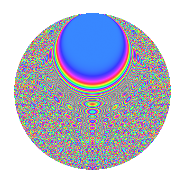# Properties

 Label 731.2.zLevel 731 Weight 2 Character orbit z Rep. character $$\chi_{731}(67,\cdot)$$ Character field $$\Q(\zeta_{42})$$ Dimension 768 Newform subspaces 1 Sturm bound 132 Trace bound 0

# Related objects

## Defining parameters

 Level: $$N$$ = $$731 = 17 \cdot 43$$ Weight: $$k$$ = $$2$$ Character orbit: $$[\chi]$$ = 731.z (of order $$42$$ and degree $$12$$) Character conductor: $$\operatorname{cond}(\chi)$$ = $$731$$ Character field: $$\Q(\zeta_{42})$$ Newform subspaces: $$1$$ Sturm bound: $$132$$ Trace bound: $$0$$

## Dimensions

The following table gives the dimensions of various subspaces of $$M_{2}(731, [\chi])$$.

Total New Old
Modular forms 816 816 0
Cusp forms 768 768 0
Eisenstein series 48 48 0

## Trace form

 $$768q - 24q^{2} - 144q^{4} - 16q^{8} - 98q^{9} + O(q^{10})$$ $$768q - 24q^{2} - 144q^{4} - 16q^{8} - 98q^{9} - 18q^{13} - 30q^{15} - 160q^{16} - 16q^{17} - 54q^{18} - 68q^{19} - 50q^{21} - 88q^{25} - 26q^{26} - 50q^{32} - 36q^{33} - 38q^{34} + 14q^{35} + 328q^{36} - 44q^{38} - 148q^{42} + 102q^{43} - 64q^{47} + 298q^{49} + 40q^{50} - 31q^{51} - 38q^{52} - 28q^{53} - 80q^{55} - 16q^{59} - 34q^{60} - 64q^{64} - 126q^{66} + 74q^{67} - 132q^{68} - 28q^{69} + 50q^{70} + 26q^{72} - 258q^{76} - 112q^{77} + 90q^{81} + 48q^{83} - 298q^{84} + 36q^{85} + 142q^{86} + 192q^{87} - 120q^{89} - 188q^{93} + 64q^{94} + 146q^{98} + O(q^{100})$$

## Decomposition of $$S_{2}^{\mathrm{new}}(731, [\chi])$$ into newform subspaces

Label Dim. $$A$$ Field CM Traces $q$-expansion
$$a_2$$ $$a_3$$ $$a_5$$ $$a_7$$
731.2.z.a $$768$$ $$5.837$$ None $$-24$$ $$0$$ $$0$$ $$0$$

## Hecke Characteristic Polynomials

There are no characteristic polynomials of Hecke operators in the database